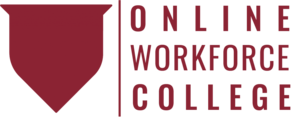#Fractions & Decimals - Skill Group Pathway is a Program

Self-paced

## Overview

A fraction is a way to express a portion of something greater. In this course, you will discover the parts that make up a fraction and how to use fractions in solving problems. You will also learn about decimal numbers and how to use them in solving problems.

By the end of this course, you will be able to

• Understand what a fraction represents
• Identify and compare fractions
• Convert and simplify fractions
• Convert between fractions, decimal numbers, and percentages
• Add, subtract, multiply, and divide fractions and decimal numbers
• Break down a word problem

Estimated completion time (hours): 3.5